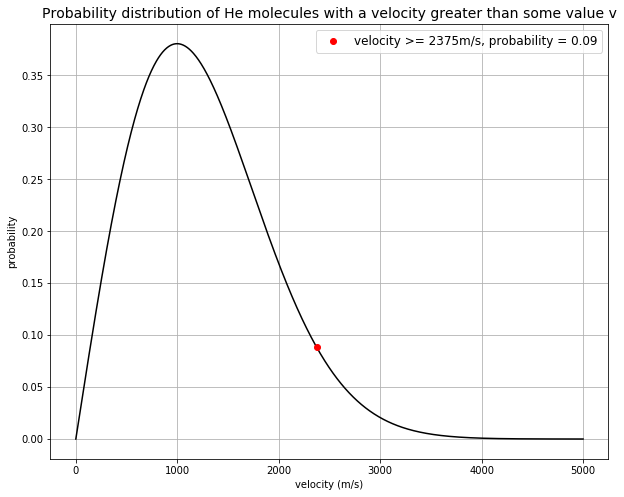# Gravity#

## Newton’s Law of Universal Gravitation#

The gravitational force $$F$$ that body $$A$$ exerts on body $$B$$ has a magnitude that is directly proportional to the mass of body $$A$$ $$(m_a)$$ and the mass of body $$B$$ $$(m_b)$$, and inversely proportional to the square of the distance between their centres $$R$$.

Mathematically:

$F=\frac{Gm_am_b}{R^2}$

where $$G$$ is the gravitational constant, equal to $$6.674\times 10^{-11}\,N\,m^2kg^{-2}$$.

Therefore, the gravitational force exerted on a mass $$m$$ by a planet of mass $$m_p$$ is:

$F=\frac{Gm_pm}{R_p^2}$

where $$m_p$$ and $$R_p$$ is the mass and radius of a planet respectively.

The local gravitational acceleration $$g$$ is:

$a=\frac{F}{m}=\frac{Gm_p}{R_p^2}=g$

In terms of density $$\rho$$:

$g=\frac{4\pi\rho R_p^3G}{3R_p^2}=\frac{4\pi\rho R_pG}{3}$

## Gravitational Potential Energy#

The definition of change in energy is that a force $$F$$ moves a body from position 1 $$R_1$$ to position 2 $$R_2$$. In the case of change in gravitational potential energy $$GPE$$ on Earth:

$F=-\frac{Gm_Em}{R^2}$

where $$m_E$$ and $$R$$ are the mass of the Earth and distance from Earth’s centre respectively,

$GPE_2-GPE_1=-\int_{R_1}^{R_2}F(R)\,dR=Gm_Em\left(\frac{1}{R_1}-\frac{1}{R_2}\right)$

Taking $$R_2$$ to be infinitely far away from the Earth:

$GPE(\infty)-GPE(R_1)=Gm_Em(\frac{1}{R_1}-0)=\frac{Gm_Em}{R_1}$

Rearranging for $$GPE(R_1)$$:

$GPE(R_1)=-\frac{Gm_Em}{R_1}$

For a body at an elevation $$h$$ close to the surface, $$GPE$$ is roughly equal to $$mgh$$.

## Escape Velocity#

To fully escape the gravitational field of a planet, the object must reach “infinity” where $$GPE=0$$. Thus, taking position 2 as infinity:

$KE(1)+GPE(R_E)=KE(2)+GPE(\infty)$

To find the minimum velocity required, we set $$KE(2)=0$$, and since $$GPE(\infty)=0$$:

$KE(1)+GPE(R_E)=0$
$\frac{mv_e^2}{2}-\frac{Gm_Em}{R_E}=0$

Rearranging for escape velocity $$v_e$$:

$v_e=\sqrt{\frac{2Gm_E}{R_E}}$

### Maxwell-Boltzmann distribution#

At absolute temperature $$T$$, the mean energy of monoatomic molecules is given by:

$\frac{3}{2}k_BT$

where $$k_B$$ is the Boltzmann’s constant equal to $$1.38\times10^{-23}\,m^2kg\,s^{-2}K^{-1}$$.

Ignoring potential energy and assuming that the energy of the monoatomic molecules is only from their kinetic energy, we can equate the above equation with the kinetic energy equation:

$\frac{mv_{mean}^2}{2}=\frac{3}{2}k_BT$

where $$m$$ is the mass of that monoatomic molecule, and $$v_{mean}$$ is the mean velocity of the monoatomic molecules at temperature $$T$$.

Rearranging for $$v_{mean}$$:

$v_{mean}=\sqrt{\frac{3k_BT}{m}}$

The probability of a molecule having a velocity higher than some value $$v$$ is given by:

$\frac{v}{v_{mean}}e^{-1.27\left(\frac{v}{v_{mean}}\right)^2}$

## Tutorial Problem 5.3#

What is the probability of Helium atoms escaping from the Moon’s surface?

Given that the mass and radius of the Moon are $$7.35\times10^{22}\,kg$$ and $$1.74\times10^6\,m$$ respectively, average surface temperature on the Moon is $$400\,K$$, and mass of a helium atom is $$6.54\times10^{-27}\,kg$$.

import numpy as np
import matplotlib.pyplot as plt

def escape(m, R, G=6.674e-11): # function for calculating escape velocity given mass and radius
return np.sqrt((2*G*m)/R)

mM = 7.35e22 # mass of Moon (kg)
RM = 1.74e6 # radius of Moon (m)

ve = escape(mM, RM)

print("Escape velocity of the Moon is %.f m/s" % (ve))

def find_vmean(m, T, kB=1.38e-23):
return np.sqrt((3*kB*T)/m)

mHe = 6.54e-27 # mass of helium atom (kg)
TM = 400 # surface temperature on the Moon (T)

vm = find_vmean(mHe, TM)

print("Mean velocity of He atoms on the Moon is %.f m/s" % (vm))

def maxwell_boltzmann(v, v_mean): # maxwell-boltzmann distribution
return v/v_mean * np.exp(-1.27*(v/v_mean)**2)

v = np.linspace(0, 5000, 1001)

prob = maxwell_boltzmann(v, vm) # array of probabilities at different velocities

# plot probability distribution

fig = plt.figure(figsize=(10,8))
plt.plot(v, prob, 'k')
plt.plot(v, prob, 'ro', label='velocity >= %.fm/s, probability = %.2f' % (v, prob))
plt.xlabel('velocity (m/s)')
plt.ylabel('probability')
plt.title('Probability distribution of He molecules with a velocity greater than some value v', fontsize=14)
plt.legend(loc='upper right', fontsize=12)
plt.grid(True)

plt.show()

Escape velocity of the Moon is 2375 m/s
Mean velocity of He atoms on the Moon is 1591 m/s